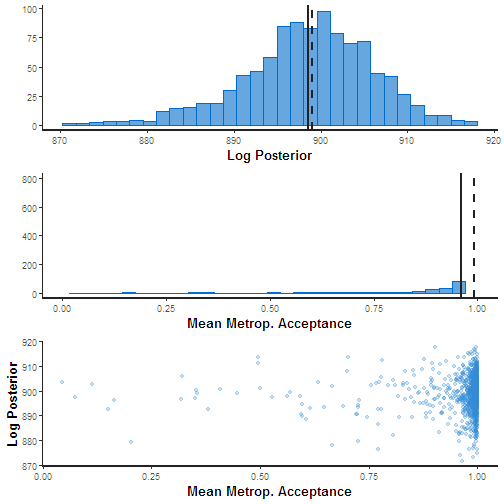# Bayesian

Jun Kang
September 4, 2019

### Coin toss

• Observed data (H, H, H, H, H, H, H, H, H, H)
• Guess the probability of coming head: 0.5? or 1.0?

### Frequentist approch (What usually has been done)

• Null hypothesis: probability of coming head is 0.5
• Hypothesis testing: Probability of observed data under null hypothesis a.k.a. p-value = ($$\frac{1}{2}^{10}$$)
• Hypothesis rejection: probability of coming head is not 0.5

### Coin toss prior probability

• The gambling dealer!!
• Guess the probability of coming head after seeing 10 continuous head: 0.5? or 1.0?

### Coin toss prior probability

• Stanford reseach gives the probability 0.51!!
• Guess the probability of coming head after seeing 10 continuous head: 0.5? 0.51? 0.6? 0.7? 1.0?

### Bayes theorem 1

$\begin{equation} \label{eq:bayes} P(\theta|\textbf{D}) = P(\theta ) \frac{P(\textbf{D} |\theta)}{P(\textbf{D})} \end{equation}$

### Bayes theorem 2

$\text{Posterior} = (\text{Prior} * \text{Likelyhood} )/\text{Normalizing constant}$

• Posterior
• Prior
• Likelyhood
• Normalizing constant

Likelyhood function

$\mathcal{L}(\theta \mid x) = p_\theta (x) = P_\theta (X=x)$

$\mathcal{L}(p_\text{H}=0.5 \mid \text{HH}) = 0.25.$

$\mathcal{L}(p_\text{H}=1.0 \mid \text{HH}) = 1.0.$

Probability distribution function

$\sum_u \operatorname{P}(X=u) = 1$

$P(\text{H} \mid p_\text{H}=0.5) = 0.5.$

$P(\text{T} \mid p_\text{H}=0.5) = 0.5.$

### Genotype calling

Genome Res. 2009. 19: 1124-1132

### Likelyhood of genotype calling

• Allele type
• Quality score
• t-th occurrence

A:0, T:3, G:0, C:0,
phred score: 30, 30, 30
Likelyhood?

### Likelyhood of genotype calling

$\text{L(Genotype T/G)|Read(T,T,T))} = (0.5*(1-0.001) + 0.5*0.001)^3$

$\text{L(Genotype T/T)|Read(T,T,T))} = (1-0.001)^3$

$\text{.}$ $\text{.}$ $\text{.}$

### Prior probability• Reference allele: G
• Homozygous SNP rate: 0.0005
• Heterozygous SNP rate: 0.001
• Ratio of transitions versus transversions: 4

### Posterior probability (incomplete)

$\text{Posterior (Genotype T/G|Read(T,T,T))}$

$= Prior (1.67*10^{-4}) * Likelyhood (0.5*(1-0.001) + 0.5*0.001)^3$

$=2.09*10^{-5}$

$\text{Posterior (Genotype T/T|Read(T,T,T))}$

$= Prior (8.33*10^{-5}) * Likelyhood((1-0.001)^3)$

$=8.31*10^{-5}$

### Normalizing constant

$P(D)=\sum_i P(D|H_i)P(H_i) \$

• Markov chain Monte Carlo (MCMC)
• WinBUGS
• JAGS
• STAN

### Three steps of Bayesian data analysis

• Full probability model
• Conditioning on obsereved data (posterior distribution)
• Evaluating the fit of the model and the implications of the resulting posterior distribution

Bayesian Data Analysis 3rd, Andrew Gelman et. al.

### Copy number and tumor purity estimation

A computational approach to distinguish somatic vs. germline origin of genomic alterations from deep sequencing of cancer specimens without a matched normal https://journals.plos.org/ploscompbiol/article?id=10.1371/journal.pcbi.1005965.

### SGZ method overview### Allele frequency table### Full probability model

\begin{aligned} r_i & \sim & N\left(log_2 \frac{pC_i+2(1-p)}{p \psi+2(1-p)}, \sigma_{ri} \right) \end{aligned}

\begin{aligned} f_i & \sim & N\left(\frac{pM_i+(1-p)}{pC_i+2(1-p)}, \sigma_{fi} \right) \end{aligned}

$\psi = \frac{\sum_{i}(l_iC_i) }{\sum_{i}(l_i)} \$

$$\\r_i\$$: Median-normalized log-ratio coverage of all exons within $$\\S_i\$$
$$\\f_i\$$: MAF of SNPs within segment $$\\S_i\$$
$$\\p\$$: Tumor purity
$$\\S_i\$$: Genomic segment
$$\\l_i\$$: Length of $$\\S_i\$$
$$\\C_i\$$: Copy number of $$\\S_i\$$
$$\\M_i\$$: Copy number of minor alleles in $$\\S_i\$$, $$\\0 \leq M_i \leq S_i\$$
$$\psi$$: Tumor ploidy of the sample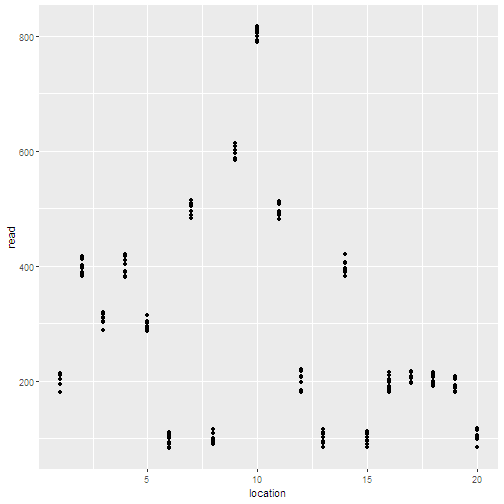### Toy data (MAF distribution)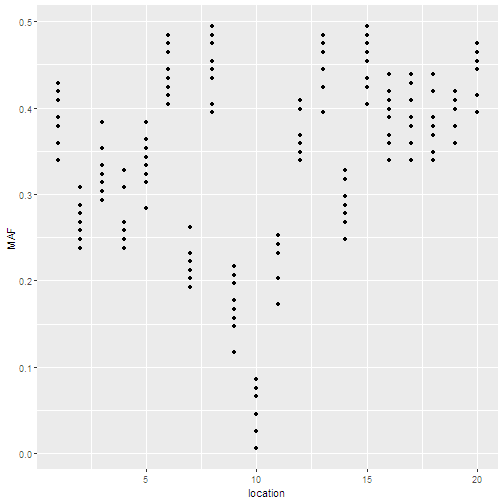### Stan code (continued)

'data {
int N;
real r[N];
real f[N];
int<lower=1> Nsub;
int<lower=1> s[N];
}'


### Stan code (continued)

'parameters {
real<lower=0.1, upper=10> cn[Nsub];
real m_logit[Nsub];
vector<lower=0>[Nsub] sigma_cn;
vector<lower=0>[Nsub] sigma_m;
real<lower=0, upper=1.0> P;
}
transformed parameters {
real m[Nsub];
real psi;
for (i in 1:Nsub){
m[i] = (0+(cn[i]/2-0)*inv_logit(m_logit[i])); //log jacobian determinant stan constraints transformation
}
psi = mean(cn);
}'


### Stan code (last)

'model {
for(i in 1:N){
r[i] ~ normal(log2((P*cn[s[i]] + 2*(1-P))/(P*psi + 2*(1-P))),sigma_cn[s[i]]);
f[i] ~ normal((P*m[s[i]]+1-P)/(P*cn[s[i]]+2*(1-P)), sigma_m[s[i]]);
}
}'


### Fit

set.seed(456278)
fit <- stan(model_code = code, data = mydata, iter = 1000,
chains = 2, control = list(adapt_delta = 0.9,
max_treedepth = 5))


SAMPLING FOR MODEL 'bbf094d9726824acefd8182f735092aa' NOW (CHAIN 1).
Chain 1:
Chain 1: Gradient evaluation took 0 seconds
Chain 1: 1000 transitions using 10 leapfrog steps per transition would take 0 seconds.
Chain 1:
Chain 1:
Chain 1: Iteration:   1 / 1000 [  0%]  (Warmup)
Chain 1: Iteration: 100 / 1000 [ 10%]  (Warmup)
Chain 1: Iteration: 200 / 1000 [ 20%]  (Warmup)
Chain 1: Iteration: 300 / 1000 [ 30%]  (Warmup)
Chain 1: Iteration: 400 / 1000 [ 40%]  (Warmup)
Chain 1: Iteration: 500 / 1000 [ 50%]  (Warmup)
Chain 1: Iteration: 501 / 1000 [ 50%]  (Sampling)
Chain 1: Iteration: 600 / 1000 [ 60%]  (Sampling)
Chain 1: Iteration: 700 / 1000 [ 70%]  (Sampling)
Chain 1: Iteration: 800 / 1000 [ 80%]  (Sampling)
Chain 1: Iteration: 900 / 1000 [ 90%]  (Sampling)
Chain 1: Iteration: 1000 / 1000 [100%]  (Sampling)
Chain 1:
Chain 1:  Elapsed Time: 1.253 seconds (Warm-up)
Chain 1:                1.252 seconds (Sampling)
Chain 1:                2.505 seconds (Total)
Chain 1:

SAMPLING FOR MODEL 'bbf094d9726824acefd8182f735092aa' NOW (CHAIN 2).
Chain 2:
Chain 2: Gradient evaluation took 0 seconds
Chain 2: 1000 transitions using 10 leapfrog steps per transition would take 0 seconds.
Chain 2:
Chain 2:
Chain 2: Iteration:   1 / 1000 [  0%]  (Warmup)
Chain 2: Iteration: 100 / 1000 [ 10%]  (Warmup)
Chain 2: Iteration: 200 / 1000 [ 20%]  (Warmup)
Chain 2: Iteration: 300 / 1000 [ 30%]  (Warmup)
Chain 2: Iteration: 400 / 1000 [ 40%]  (Warmup)
Chain 2: Iteration: 500 / 1000 [ 50%]  (Warmup)
Chain 2: Iteration: 501 / 1000 [ 50%]  (Sampling)
Chain 2: Iteration: 600 / 1000 [ 60%]  (Sampling)
Chain 2: Iteration: 700 / 1000 [ 70%]  (Sampling)
Chain 2: Iteration: 800 / 1000 [ 80%]  (Sampling)
Chain 2: Iteration: 900 / 1000 [ 90%]  (Sampling)
Chain 2: Iteration: 1000 / 1000 [100%]  (Sampling)
Chain 2:
Chain 2:  Elapsed Time: 1.23 seconds (Warm-up)
Chain 2:                1.247 seconds (Sampling)
Chain 2:                2.477 seconds (Total)
Chain 2:


### Posterior of copy number

plot(fit, pars = 'cn')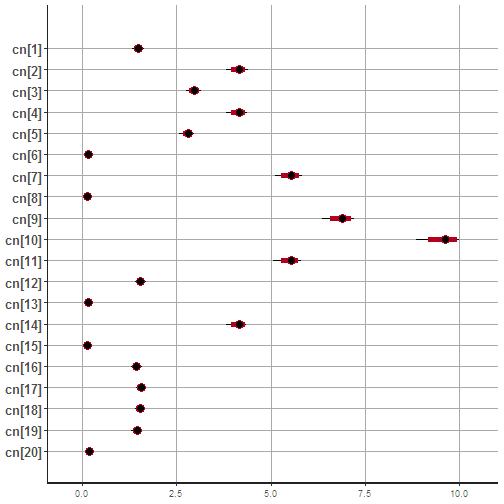### Posterior of minor allele copy number

plot(fit, pars = 'm')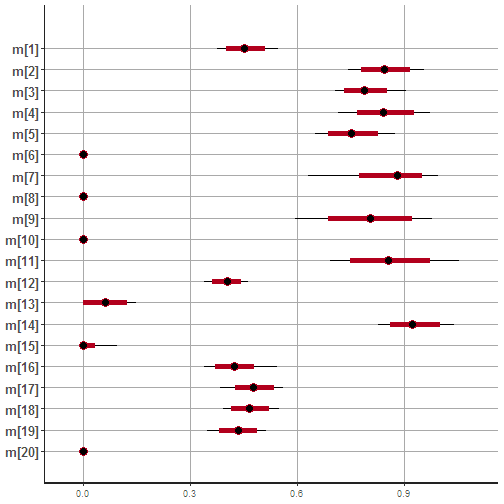### Posterior of tumor purity

post <- extract(fit)

hist(post\$P,
main = paste("Tumor purity"),
ylab = '')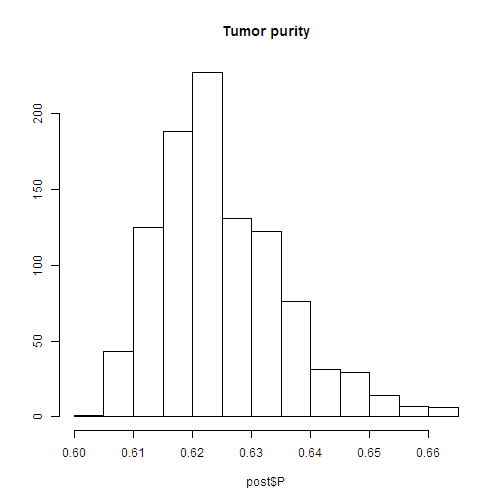### Trace Plot

traceplot(fit, pars = 'cn')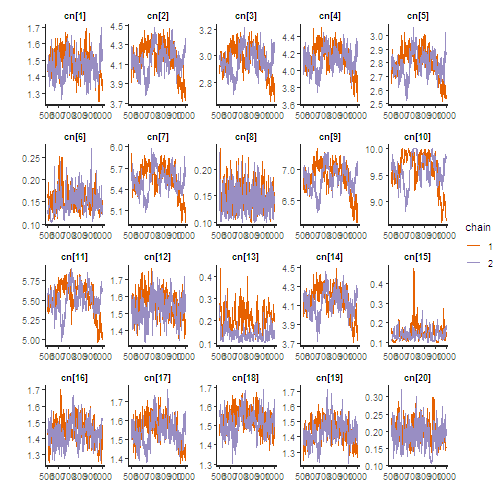### Diagnostic Plot

stan_diag(fit)